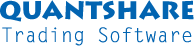#twitter-follow { border: 0;position: fixed; top: 240px; left:0;} #live-support { border: 0;position: fixed; top: 370px; left:0;} #knowledge-base { border: 0;position: fixed; top: 170px; left:0;}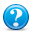SUM for the past X days with intraday time series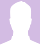umair 2013-03-10 21:40:30 a = Crossindicator(close); b = hour() == 16; c = iff(b==1,a,0); d = sum(c,ref(day(),9)); Plot(d, "Crossindicator", colorBlack, chartLine, StyleSymbolNone); hi i'm working with intraday data and i'm trying to get my values for "c" summed for the past X amount of days. d= sum(c, for the past 9 days); Any idea why my formula is not working>> THXumair 2013-03-10 21:45:230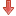No solutions for this either; a = Crossindicator(close); b = hour() == 16; c = iff(b==1,a,0); d = sum(c,barssince(day() != ref(day(), 2))); Plot(d, "Crossindicator", colorBlack, chartLine, StyleSymbolNone);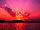QuantShare 2013-03-11 09:42:460The calculation of "c" is based on intraday data. Would you like to sum all past "c" values for the last X days (based on intraday data)?umair 2013-03-11 17:01:250Yes, the calculation for C is based on intraday data. My crossindicator counts how many a cross occured throughout the day. So by matching it with "B" gives me the last value for crossindicator (i.e the max value). So to answer your second question, yes, I would like to sum up the value at 1600 for cross indicator for the past X days. "X days" should be dynamic in a sense that If X = 2, it sums up C for the past two days. I understand that I can use sum(c,300) but that only sums up X for the past 300 days. There should be a way that I can create a logic that dynamically changes the number of days I am looking at. For example: day1 C = 2 day2 C= 4 day3 C =5 so, sum(c,for the past 3 DAYS rather than bars)= 11QuantShare 2013-03-11 22:44:540You are using intraday data, so using "sum(c, 300)" will sums up the past 300 bars (not days). If you are using hourly data, you can multiply the number of bars per the number of hours in a trading day to get the number of days. If you need dynamic past values then use the "sumif" function instead. Unlike "sum", this one accepts dynamic values.umair 2013-03-12 04:11:150"You are using intraday data, so using "sum(c, 300)" will sums up the past 300 bars (not days)." // yea i know...... I dont know what you mean by ''dynamic.'' That word can have several meanings but the context in which it's used can be important. So please refer back to my previous replies to understand the context I'm using that word in. Back to the screener: Here's what I've came up with. It's the closest I have been to the solution that I am looking for but there is something still wrong as the system does not differentiate between different days a = Crossindicator(close); b = hour() == 16; e = iff(b==1,a,0); days = 2; bars = 391*days; d = sum(e,bars); So the above should sum up 391*2 (the number of bars in two days) the values of E the values of E for the past two days are: day0 =17 day1= 5 day2= 10 So the solution should be 15 (sum of the past TWO DAYS) The results given by the above are: day0 =0 day1=0 day2=27 But then toward the end of the 2nd day the value changes to 30 Any idea why? I would appreciate if you can give me a solution (logic) this time, it seems i'm spending way too much time on this as it should be a simple logic to program!umair 2013-03-12 06:24:070Ok, another thing i just noticed is that if i run the above filter as a screener and add a column for D it actually gives me the correct results! sum for past 2 days is 15 and sum for past 3 days is 32. So i'm not sure why when I PLOT this on a graph i'm getting different results.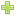Get more messages
No more messages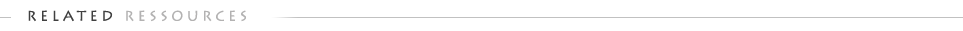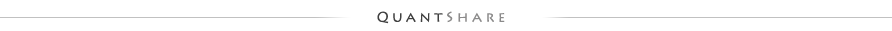Support Contact Us Trading Forum How-to Lessons Manual Company About Us Privacy Terms of Use Copyright © 2022 QuantShare.com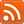Trading financial instruments, including foreign exchange on margin, carries a high level of risk and is not suitable for all investors. The high degree of leverage can work against you as well as for you. Before deciding to invest in financial instruments or foreign exchange you should carefully consider your investment objectives, level of experience, and risk appetite. The possibility exists that you could sustain a loss of some or all of your initial investment and therefore you should not invest money that you cannot afford to lose. You should be aware of all the risks associated with trading and seek advice from an independent financial advisor if you have any doubts.